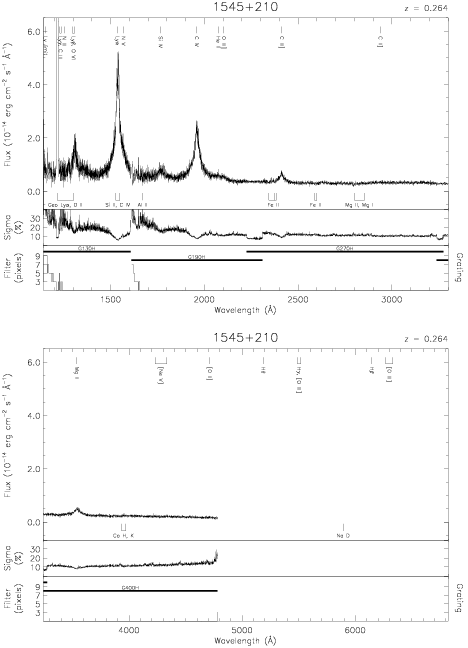Download dataset
TELESCOP= 'HST     '
INSTRUME= 'FOS     '
EQUINOX =               2000.0
DATE-OBS= '1992-04-08T01:40:40'
RA_PNT  =       236.9313750000
DEC_PNT =        20.8712222222
PA_PNT  =       120.4390231571
OBJECT  = '1545+210'
ROOTNM01= 'Y0PE0C02T'
GRNDMD01= 'SPECTROSCOPY'
DETECT01= 'BLUE    '
APERID01= 'A-1     '
FGWAID01= 'H13     '
ROOTNM02= 'Y0PE0C03T'
GRNDMD02= 'SPECTROSCOPY'
DETECT02= 'BLUE    '
APERID02= 'A-1     '
FGWAID02= 'H13     '
ROOTNM03= 'Y0PE0D02T'
GRNDMD03= 'SPECTROSCOPY'
DETECT03= 'AMBER   '
APERID03= 'A-1     '
FGWAID03= 'H19     '
ROOTNM04= 'Y0PE0D03T'
GRNDMD04= 'SPECTROSCOPY'
DETECT04= 'AMBER   '
APERID04= 'A-1     '
FGWAID04= 'H27     '
ROOTNM05= 'Y0PE0D04T'
GRNDMD05= 'SPECTROSCOPY'
DETECT05= 'AMBER   '
APERID05= 'A-1     '
FGWAID05= 'H40     '